How to use current row number

To arrive at the current row number we need to use the ROW function. We can use Row function to provide the reference in the formula.

Lets understand what this function is and how it works.

ROWS function is used to return the rows number of a cell reference or array.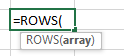Now we will learn about how to use the Row function along with another formulas.

We have data about fruits with their respective quantity sold. Lets see how we can find out the 3 smallest sold quantity and 3 largest sold quantity.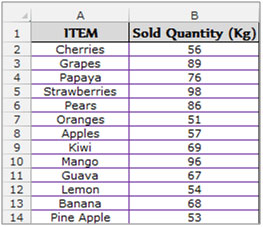We have to use the “Small” and “Large” function along with the “Row” function.
Firstly we have to understand the “Small” and “Large” functions individually.

SMALL function we used to return the k-th smallest value from a range.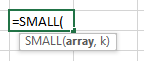Example:The seventh smallest value

LARGE function is used for return the k-th largest value in a data set.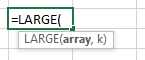Example:The seventh largest value.

To return the 3 smallest sold quantity follow the below mentioned steps:-

• Select the cell A18 and write the formula.
• =SMALL (\$B\$2:\$B\$14, ROWS (A\$18:A18)) and press enter on the keyboard.
• Copy the same formula and paste into cells A19 and A20.
• The function will return the 3 smallest sold quantity.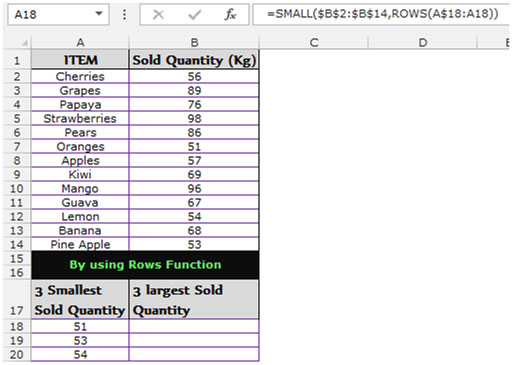To return the 3 largest sold quantity follow the below mentioned steps:-

• Select the cell B18 and write the formula.
• =LARGE (\$B\$2:\$B\$14, ROWS (B\$18:B18)) and press enter on the keyboard.
• Copy the same formula and paste into cells B19 and B20.
• The function will return the 3 largest sold quantity.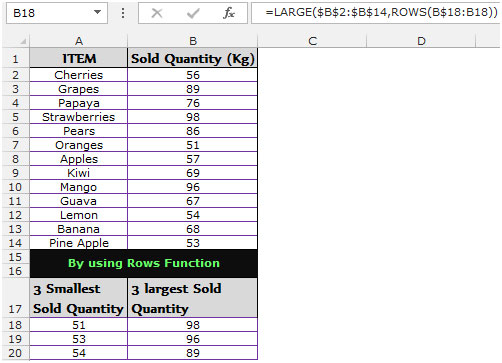In the above example we used the Rows function along with the Large and Small to get the row number, It will provide us the row no for the first largest and smallest value as well as for the second and third largest and smallest values. This combination of functions is useful when you have to perform analysis and arrive at the Top 3 or Top 5 or Top 10 out of the data provided.We would love to hear from you, do let us know how we can improve, complement or innovate our work and make it better for you. Write us at info@exceltip.com

## Users are saying about us...

1.Great example except the explanation needs to be clarified to point out the difference of the functions ROW and ROWS. Your text explanation depicts the ROW function, but your formula uses the ROWS function.

Terms and Conditions of use

The applications/code on this site are distributed as is and without warranties or liability. In no event shall the owner of the copyrights, or the authors of the applications/code be liable for any loss of profit, any problems or any damage resulting from the use or evaluation of the applications/code.